Foxtable(狐表)用户栏目专家坐堂 → 动态表的表名问题

共有450人关注过本帖树形打印复制链接

# 主题：动态表的表名问题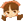1楼 | 信息 | 搜索 | 邮箱 | 主页 | UC加好友发短信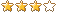我想把动态生成的表中手工录入的当前局成绩保存到比赛积分表，结果报错：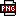此主题相关图片如下：qq图片20211113210152.png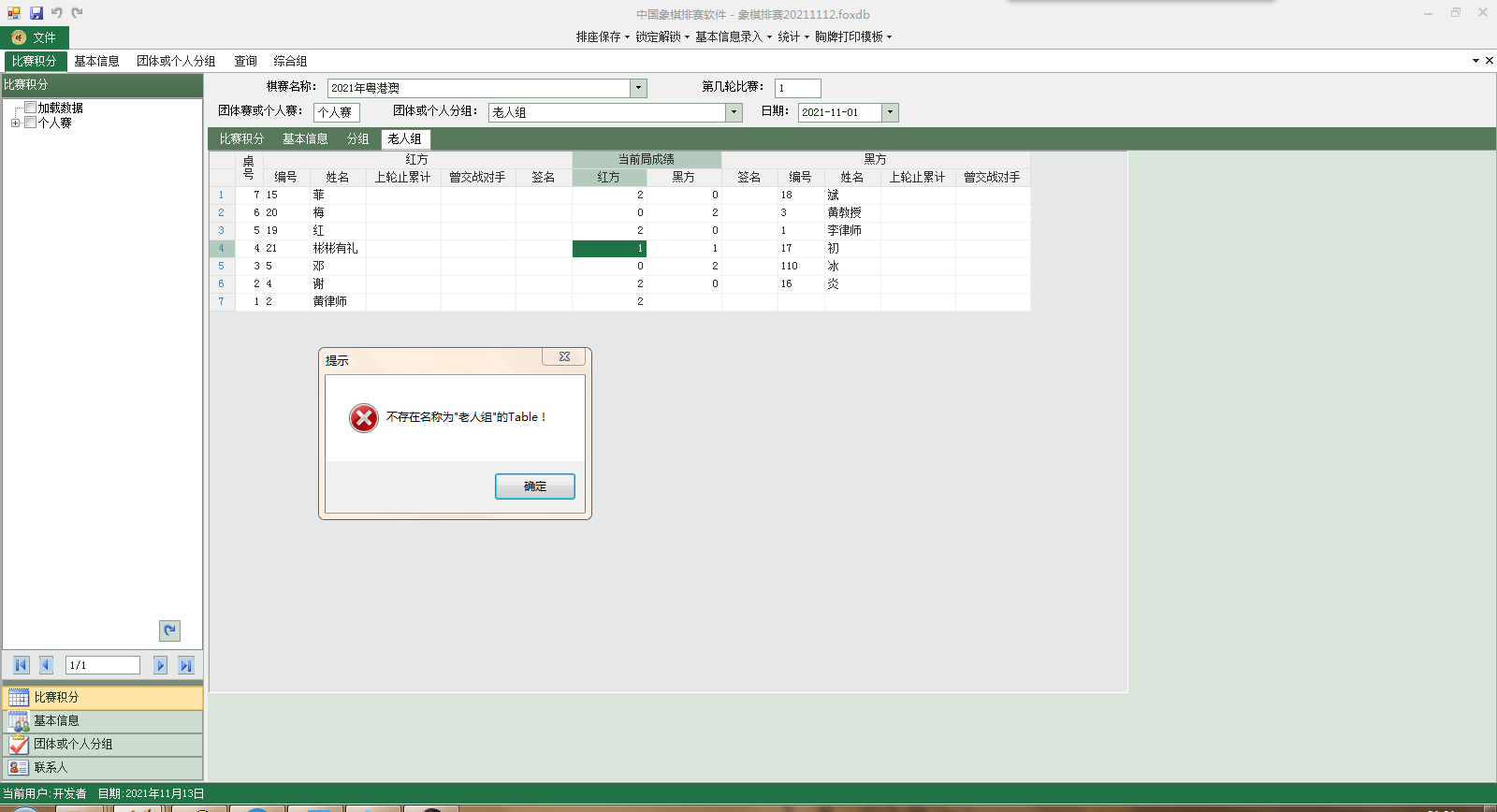此主题相关图片如下：qq图片20211113210234.png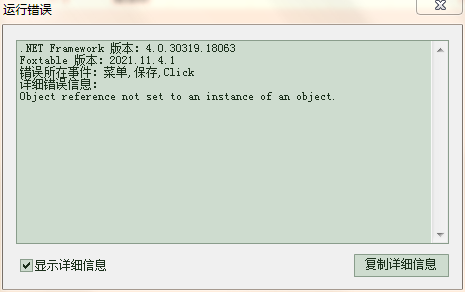我想应是这个动态表合成名称不对：Dim qsmc As WinForm.ComboBox = Forms("主窗口").Controls("棋赛名称")Dim di1 As String = qsmc.ValueDim m As WinForm.TextBox = Forms("主窗口").Controls("第几轮")Dim di2 As String = m.ValueDim bsrq As WinForm.DateTimePicker = Forms("主窗口").Controls("rq")Dim di3 As Date = bsrq.ValueDim bslx As WinForm.TextBox = Forms("主窗口").Controls("bslx")Dim lx As String = bslx.ValueDim fz As WinForm.DropDownBox = Forms("主窗口").Controls("fenzhu")Dim Str As String = fz.ValueDim tc As WinForm.TabControl = Forms("主窗口").Controls("TabControl1")Dim dt As Table = Tables(tc.SelectedPage.Name)tc.SelectedPage.Name = StrFor Each nr As Row In dt.Rows    Dim dr1 As DataRow = DataTables("比赛积分").SQLfind("[棋赛名称] = '" & di1 & "' And [第几轮比赛] = '" & di2 & "' And [团体赛或个人赛] = '" & lx & "' And [所属团体或个人分组] = '" & Str & "' And [编号] = '" & nr("红方_编号") & "' And [姓名] = '" & nr("红方_姓名") & "'")    If dr1 IsNot Nothing Then        If nr("桌号") > 0  Then            dr1("积分") = nr("当前局成绩_红方")            If di2 = 1 Then                dr1("红黑方") = "红方"                dr1("本轮止累计积分") = nr("当前局成绩_红方")                dr1("本次止曾交战对手累计积分") = nr("当前局成绩_黑方")            ElseIf di2 >= 2 Then                dr1("本轮止累计积分") = nr("红方_上轮止累计积分") + nr("当前局成绩_红方")                dr1("本次止曾交战对手累计积分") = nr("红方_曾交战对手累计积分") + nr("当前局成绩_黑方")            End If        ElseIf nr.IsNull("桌号") And dr1("编号") = nr("红方_编号") Then            dr1("红黑方") = "红方"            dr1("积分") = 0            If di2 = 1 Then                dr1("本轮止累计积分") = nr("当前局成绩_红方")                dr1("本次止曾交战对手累计积分") = nr("当前局成绩_黑方")            ElseIf di2 >= 2 Then                dr1("本轮止累计积分") = nr("红方_上轮止累计积分") + nr("当前局成绩_红方")                dr1("本次止曾交战对手累计积分") = nr("红方_曾交战对手累计积分") + nr("当前局成绩_黑方")            End If        End If        dr1.Save    End If    Dim dr2 As DataRow = DataTables("比赛积分").SQLfind("[棋赛名称] = '" & di1 & "' And [第几轮比赛] = '" & di2 & "' And [团体赛或个人赛] = '" & lx & "' And [所属团体或个人分组] = '" & Str & "' And [编号]='" & nr("黑方_编号") & "'And [姓名] = '" & nr("红方_姓名") & "'")    If dr2 IsNot Nothing Then        If di3 <> Nothing Then            dr2("日期") = di3        End If        If nr("桌号") > 0 Then            dr2("红黑方") = "黑方"            dr2("积分") = nr("当前局成绩_黑方")            If di2 = 1 Then                dr2("本轮止累计积分") = nr("当前局成绩_黑方")                dr2("本次止曾交战对手累计积分") = nr("当前局成绩_红方")            ElseIf di2 >= 2 Then                dr2("本轮止累计积分") = nr("黑方_上轮止累计积分") + nr("当前局成绩_黑方")                dr2("本次止曾交战对手累计积分") = nr("黑方_曾交战对手累计积分") + nr("当前局成绩_黑方")            End If        ElseIf nr.IsNull("桌号") And dr2("编号") = nr("黑方_编号") Then            dr2("红黑方") = "黑方"            dr2("积分") = 0            If di2 = 1 Then                dr2("本轮止累计积分") = nr("当前局成绩_黑方")                dr2("本次止曾交战对手累计积分") = nr("当前局成绩_红方")            ElseIf di2 >= 2 Then                dr2("本轮止累计积分") = nr("黑方_上轮止累计积分") + nr("当前局成绩_黑方")                dr2("本次止曾交战对手累计积分") = nr("黑方_曾交战对手累计积分") + nr("当前局成绩_红方")            End If        End If        dr2.Save    End IfNextDim drs As List(of DataRow) = DataTables("比赛积分").SQLSelect("[棋赛名称] = '" & di1 & "'And [第几轮比赛] = '" & di2 & "' And [所属团体或个人分组] = '" & Str & "'","","本轮止累计积分 Desc,本次止曾交战对手累计积分 Desc")If drs IsNot Nothing Then    Dim i1 As Integer = 1    For n As Integer = 0 To drs.Count - 1 '遍历所有行        If n > 0 Then            drs(n)("名次") = 1        ElseIf n > 0 And (drs(n)("本轮止累计积分") = drs(n-1)("本轮止累计积分") AndAlso drs(n)("本次止曾交战对手累计积分") > drs(n-1)("本次止曾交战对手累计积分") Or (drs(n)("本轮止累计积分") > drs(n-1)("本轮止累计积分"))) Then            i1 = i1 + 1            drs(n)("名次") = i1        Else            i1 = i1            drs(n)("名次") = i1        End If    NextEnd IfDataTables("比赛积分").SQLUpdate(drs)DataTables("比赛积分").load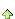2楼 | 信息 | 搜索 | 邮箱 | 主页 | UC加好友发短信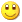Post By：2021/11/14 11:15:00 [只看该作者]

.NET Framework 版本：4.0.30319.18063
Foxtable 版本：2021.11.12.11

Value cannot be null.
Parameter name: key
 下载信息  [文件大小：   下载次数： ]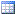点击浏览该文件:象棋排赛222.foxdb

Dim qsmc As WinForm.ComboBox = Forms("主窗口").Controls("棋赛名称")
Dim di1 As String = qsmc.Value
Dim m As WinForm.TextBox = Forms("主窗口").Controls("第几轮")
Dim di2 As String = m.Value
Dim bsrq As WinForm.DateTimePicker = Forms("主窗口").Controls("rq")
Dim di3 As Date = bsrq.Value
Dim bslx As WinForm.TextBox = Forms("主窗口").Controls("bslx")
Dim lx As String = bslx.Value
Dim fz As WinForm.DropDownBox = Forms("主窗口").Controls("fenzhu")
Dim Str As String = fz.Value
For Each nr As Row In Tables(Vars("Str")).Rows
Dim dr1 As DataRow = DataTables("比赛积分").SQLfind("[团体赛或个人赛] = '" & lx & "'And [棋赛名称] = '" & di1 & "' And [第几轮比赛] = '" & di2 & "' And [所属团体或个人分组] = '" & Str & "' And [编号] = '" & nr("红方_编号") & "' And [姓名] = '" & nr("红方_姓名") & "'")
If dr1 IsNot Nothing Then
If nr("桌号") > 0  Then
dr1("积分") = nr("当前局成绩_红方")
If di2 = 1 Then
dr1("红黑方") = "红方"
dr1("本轮止累计积分") = nr("当前局成绩_红方")
dr1("本次止曾交战对手累计积分") = nr("当前局成绩_黑方")
ElseIf di2 >= 2 Then
dr1("本轮止累计积分") = nr("红方_上轮止累计积分") + nr("当前局成绩_红方")
dr1("本次止曾交战对手累计积分") = nr("红方_曾交战对手累计积分") + nr("当前局成绩_黑方")
End If
ElseIf nr.IsNull("桌号") And dr1("编号") = nr("红方_编号") Then
dr1("红黑方") = "红方"
dr1("积分") = 0
If di2 = 1 Then
dr1("本轮止累计积分") = nr("当前局成绩_红方")
dr1("本次止曾交战对手累计积分") = nr("当前局成绩_黑方")
ElseIf di2 >= 2 Then
dr1("本轮止累计积分") = nr("红方_上轮止累计积分") + nr("当前局成绩_红方")
dr1("本次止曾交战对手累计积分") = nr("红方_曾交战对手累计积分") + nr("当前局成绩_黑方")
End If
End If
dr1.Save
End If
Dim dr2 As DataRow = DataTables("比赛积分").SQLfind("[团体赛或个人赛] = '" & lx & "' And [棋赛名称] = '" & di1 & "' And [第几轮比赛] = '" & di2 & "' And [所属团体或个人分组] = '" & Str & "' And [编号]='" & nr("黑方_编号") & "'And [姓名] = '" & nr("黑方_姓名") & "'")
If dr2 IsNot Nothing Then
If di3 <> Nothing Then
dr2("日期") = di3
End If
If nr("桌号") > 0 Then
dr2("红黑方") = "黑方"
dr2("积分") = nr("当前局成绩_黑方")
If di2 = 1 Then
dr2("本轮止累计积分") = nr("当前局成绩_黑方")
dr2("本次止曾交战对手累计积分") = nr("当前局成绩_红方")
ElseIf di2 >= 2 Then
dr2("本轮止累计积分") = nr("黑方_上轮止累计积分") + nr("当前局成绩_黑方")
dr2("本次止曾交战对手累计积分") = nr("黑方_曾交战对手累计积分") + nr("当前局成绩_黑方")
End If
ElseIf nr.IsNull("桌号") And dr2("编号") = nr("黑方_编号") Then
dr2("红黑方") = "黑方"
dr2("积分") = 0
If di2 = 1 Then
dr2("本轮止累计积分") = nr("当前局成绩_黑方")
dr2("本次止曾交战对手累计积分") = nr("当前局成绩_红方")
ElseIf di2 >= 2 Then
dr2("本轮止累计积分") = nr("黑方_上轮止累计积分") + nr("当前局成绩_黑方")
dr2("本次止曾交战对手累计积分") = nr("黑方_曾交战对手累计积分") + nr("当前局成绩_红方")
End If
End If
dr2.Save
End If
Next
Dim drs As List(of DataRow) = DataTables("比赛积分").SQLSelect("[团体赛或个人赛] = '" & lx & "' And [棋赛名称] = '" & di1 & "'And [第几轮比赛] = '" & di2 & "' And [所属团体或个人分组] = '" & Str & "'","","本轮止累计积分 Desc,本次止曾交战对手累计积分 Desc")
If drs IsNot Nothing Then
Dim i1 As Integer = 1
For n As Integer = 0 To drs.Count - 1 '遍历所有行
If n > 0 Then
drs(n)("名次") = 1
ElseIf n > 0 And (drs(n)("本轮止累计积分") = drs(n-1)("本轮止累计积分") AndAlso drs(n)("本次止曾交战对手累计积分") > drs(n-1)("本次止曾交战对手累计积分") Or (drs(n)("本轮止累计积分") > drs(n-1)("本轮止累计积分"))) Then
i1 = i1 + 1
drs(n)("名次") = i1
Else
i1 = i1
drs(n)("名次") = i1
End If
Next
End If
DataTables("比赛积分").SQLUpdate(drs)
[此贴子已经被作者于2021/11/14 16:46:28编辑过]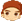3楼 | 信息 | 搜索 | 邮箱 | 主页 | UC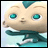加好友发短信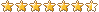Post By：2021/11/14 20:51:00 [只看该作者]

 如果是副本，窗口表名称应该是：http://www.foxtable.com/webhelp/topics/1906.htm4楼 | 信息 | 搜索 | 邮箱 | 主页 | UC加好友发短信Post By：2021/11/14 21:26:00 [只看该作者]

 怎么判断动态表是否存在？Dim tbl As WinForm.Table = Forms("主窗口").Controls("Table" & "'" & Str & "'")If DataTables.Contains(Vars("Str")) Then    For Each nr As Row In Tables("主窗口_'" & Str & "'").Rows5楼 | 信息 | 搜索 | 邮箱 | 主页 | UC加好友发短信Post By：2021/11/14 21:37:00 [只看该作者]

 如果要判断datatable，就是上面的用法如果要判断窗口表table，http://www.foxtable.com/webhelp/topics/0437.htmIf Tables.Contains(Vars("Str")) Then6楼 | 信息 | 搜索 | 邮箱 | 主页 | UC加好友发短信Post By：2021/11/14 21:54:00 [只看该作者]

 把代码改为这样，还是一样报错！.NET Framework 版本：4.0.30319.18063Foxtable 版本：2021.11.16.11错误所在事件：菜单,保存,Click详细错误信息：Value cannot be null.Parameter name: keyDim qsmc As WinForm.ComboBox = Forms("主窗口").Controls("棋赛名称")Dim di1 As String = qsmc.ValueDim m As WinForm.TextBox = Forms("主窗口").Controls("第几轮")Dim di2 As String = m.ValueDim bsrq As WinForm.DateTimePicker = Forms("主窗口").Controls("rq")Dim di3 As Date = bsrq.ValueDim bslx As WinForm.TextBox = Forms("主窗口").Controls("bslx")Dim lx As String = bslx.ValueDim fz As WinForm.DropDownBox = Forms("主窗口").Controls("fenzhu")Dim Str As String = fz.ValueIf Tables.Contains(Vars("Str")) Then    For Each nr As Row In Tables("主窗口_'" & Str & "'").Rows        Dim dr1 As DataRow = DataTables("比赛积分").SQLfind("[团体赛或个人赛] = '" & lx & "'And [棋赛名称] = '" & di1 & "' And [第几轮比赛] = '" & di2 & "' And [所属团体或个人分组] = '" & Str & "' And [编号] = '" & nr("红方_编号") & "' And [姓名] = '" & nr("红方_姓名") & "'")        If dr1 IsNot Nothing Then            If nr("桌号") > 0  Then                dr1("积分") = nr("当前局成绩_红方")                If di2 = 1 Then                    dr1("红黑方") = "红方"                    dr1("本轮止累计积分") = nr("当前局成绩_红方")                    dr1("本次止曾交战对手累计积分") = nr("当前局成绩_黑方")                ElseIf di2 >= 2 Then                    dr1("本轮止累计积分") = nr("红方_上轮止累计积分") + nr("当前局成绩_红方")                    dr1("本次止曾交战对手累计积分") = nr("红方_曾交战对手累计积分") + nr("当前局成绩_黑方")                End If            ElseIf nr.IsNull("桌号") And dr1("编号") = nr("红方_编号") Then                dr1("红黑方") = "红方"                dr1("积分") = 0                If di2 = 1 Then                    dr1("本轮止累计积分") = nr("当前局成绩_红方")                    dr1("本次止曾交战对手累计积分") = nr("当前局成绩_黑方")                ElseIf di2 >= 2 Then                    dr1("本轮止累计积分") = nr("红方_上轮止累计积分") + nr("当前局成绩_红方")                    dr1("本次止曾交战对手累计积分") = nr("红方_曾交战对手累计积分") + nr("当前局成绩_黑方")                End If            End If            dr1.Save        End If        Dim dr2 As DataRow = DataTables("比赛积分").SQLfind("[团体赛或个人赛] = '" & lx & "' And [棋赛名称] = '" & di1 & "' And [第几轮比赛] = '" & di2 & "' And [所属团体或个人分组] = '" & Str & "' And [编号]='" & nr("黑方_编号") & "'And [姓名] = '" & nr("黑方_姓名") & "'")        If dr2 IsNot Nothing Then            If di3 <> Nothing Then                dr2("日期") = di3            End If            If nr("桌号") > 0 Then                dr2("红黑方") = "黑方"                dr2("积分") = nr("当前局成绩_黑方")                If di2 = 1 Then                    dr2("本轮止累计积分") = nr("当前局成绩_黑方")                    dr2("本次止曾交战对手累计积分") = nr("当前局成绩_红方")                ElseIf di2 >= 2 Then                    dr2("本轮止累计积分") = nr("黑方_上轮止累计积分") + nr("当前局成绩_黑方")                    dr2("本次止曾交战对手累计积分") = nr("黑方_曾交战对手累计积分") + nr("当前局成绩_黑方")                End If            ElseIf nr.IsNull("桌号") And dr2("编号") = nr("黑方_编号") Then                dr2("红黑方") = "黑方"                dr2("积分") = 0                If di2 = 1 Then                    dr2("本轮止累计积分") = nr("当前局成绩_黑方")                    dr2("本次止曾交战对手累计积分") = nr("当前局成绩_红方")                ElseIf di2 >= 2 Then                    dr2("本轮止累计积分") = nr("黑方_上轮止累计积分") + nr("当前局成绩_黑方")                    dr2("本次止曾交战对手累计积分") = nr("黑方_曾交战对手累计积分") + nr("当前局成绩_红方")                End If            End If            dr2.Save        End If    NextEnd IfDim drs As List(of DataRow) = DataTables("比赛积分").SQLSelect("[团体赛或个人赛] = '" & lx & "' And [棋赛名称] = '" & di1 & "'And [第几轮比赛] = '" & di2 & "' And [所属团体或个人分组] = '" & Str & "'","","本轮止累计积分 Desc,本次止曾交战对手累计积分 Desc")If drs IsNot Nothing Then    Dim i1 As Integer = 1    For n As Integer = 0 To drs.Count - 1 '遍历所有行        If n > 0 Then            drs(n)("名次") = 1        ElseIf n > 0 And (drs(n)("本轮止累计积分") = drs(n-1)("本轮止累计积分") AndAlso drs(n)("本次止曾交战对手累计积分") > drs(n-1)("本次止曾交战对手累计积分") Or (drs(n)("本轮止累计积分") > drs(n-1)("本轮止累计积分"))) Then            i1 = i1 + 1            drs(n)("名次") = i1        Else            i1 = i1            drs(n)("名次") = i1        End If    NextEnd IfDataTables("比赛积分").SQLUpdate(drs)DataTables("比赛积分").load7楼 | 信息 | 搜索 | 邮箱 | 主页 | UC加好友发短信Post By：2021/11/14 22:17:00 [只看该作者]

 学会调试msgbox(Vars("Str"))If Tables.Contains(Vars("Str")) Thenmsgbox("主窗口_'" & Str & "'")    For Each nr As Row In Tables("主窗口_'" & Str & "'").Rows8楼 | 信息 | 搜索 | 邮箱 | 主页 | UC加好友发短信Post By：2021/11/14 22:35:00 [只看该作者]

 msgbox(Vars("Str"))弹出的窗口为空，证明这样写错吧？Dim fz As WinForm.DropDownBox = Forms("主窗口").Controls("fenzhu")Dim Str As String = fz.Value控件已经选了值，合成表就没东西。 [此贴子已经被作者于2021/11/14 22:50:00编辑过]9楼 | 信息 | 搜索 | 邮箱 | 主页 | UC加好友发短信Post By：2021/11/14 22:44:00 [只看该作者]

 如果Vars("Str")这个全局变量是有用的，自己找找为什么会是空值。注意Vars("Str")和Dim Str As String不是一回事10楼 | 信息 | 搜索 | 邮箱 | 主页 | UC加好友发短信Post By：2021/11/14 22:56:00 [只看该作者]

 我就是不知当Table("成人组")、Table("女子组")、Table("少年组")、Table("男子组")、Table("老人组")、Table("甲组")、Table("乙组")......这字符是变量应自成么合成为表名称。总数 36 1 2 3 4 下一页